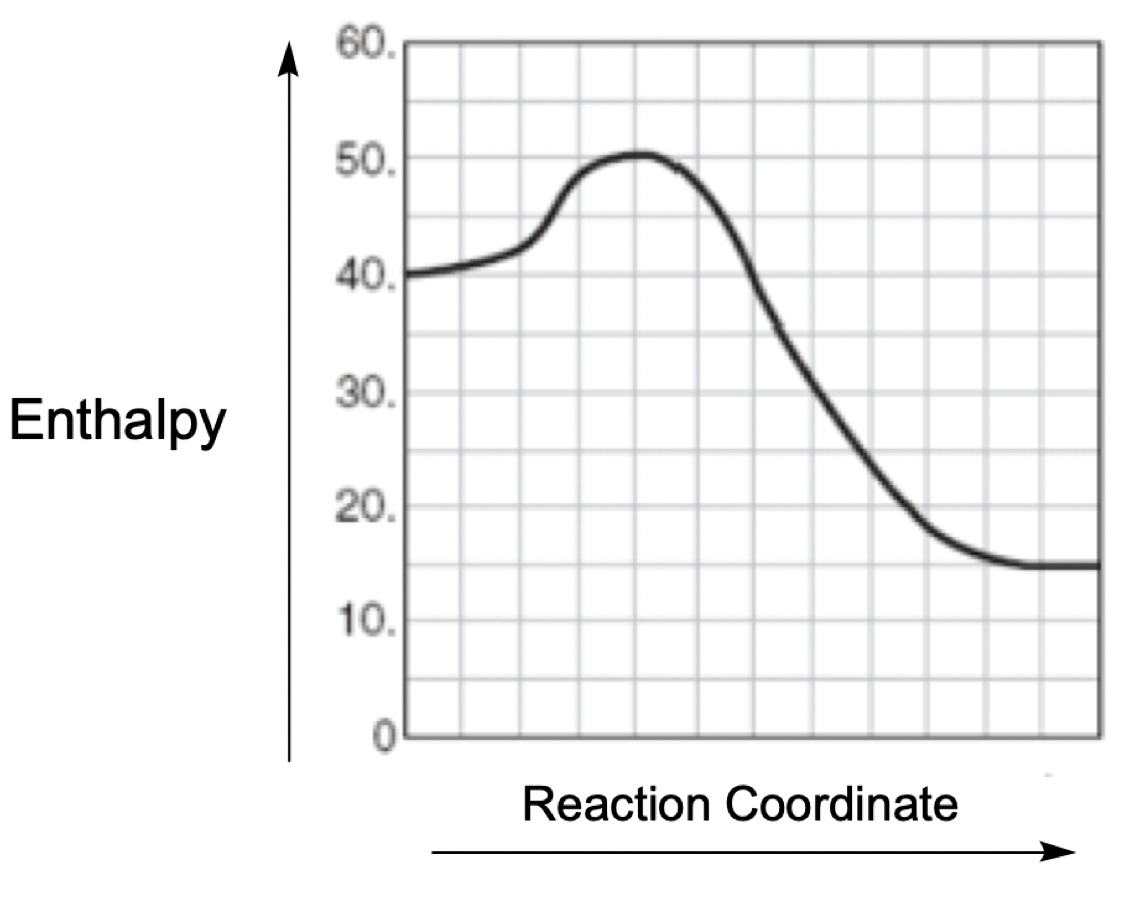## General Chemistry

Learn the toughest concepts covered in Chemistry with step-by-step video tutorials and practice problems by world-class tutors

15. Chemical Kinetics

# Catalyst

Catalyst is a substance that increases the rate of reaction by lowering the Activation Energy (Ea) of a reaction.

Catalyzed vs Uncatalyzed Reactions
1
concept

## Catalyst Concept 11m
Play a video:
a catalyst can be seen as any substance that increases the rate of reaction by decreasing your energy of activation and also not being consumed in the process. Here we have a catalyzed versus an uncapped allies reaction being displayed in terms of an energy diagram. Remember, in our energy diagram, we have our reactions in the beginning, our products at the end. The difference between the two lines represents the overall change in energy of the chemical reaction. And then if we take a look, here we have at the top of this First Hill is our transition state for it. And at the top of this hill is our transition state. The difference between the transition states and the reactant line gives us our activation energy. Remember, a catalyst lowers your energy of activation. If we take a look at the first red curve, we can see that it's activation. Energy is pretty high, so it's safe to assume that this is the energy of activation for a new catalyzed reaction. So here we'd say that this is e a uncapped. Now, when we add a catalyst, the energy of activation is expected to go down. So the line and blue represents the addition of our catalyst. So this would be activation energy cap for catalyzed. So remember, the height of the activation energy has a direct impact on the speed of the reaction, the higher the activation energy, the longer it takes from my reactant to basically climb and scale this hill to slide down and become a product. So the red line here we say something higher up in higher activation energy. It would take longer for us to make our products the catalyzed one. Since the activation energy is smaller, we expect the reactions to have an easier time getting up this hill and sliding down to make our products. So keep in mind, this is what a catalyst does. It lowers our energy of activation so that our reactions can more easily become products.
2
example

## Catalyst Example 12m
Play a video:
Hey everyone. So in this example question it says a certain reaction has an entropy value of negative killed jules and an activation energy of 40 killer jewels. A catalyst is found that lowers the activation energy of the reaction by 10 kg what is the total difference in energy between the products and the transition state? Alright, so they're telling us here are the values So this is delta H. And we can say here, delta also can fill in And it's negative 20. So let's say that where our reactant start here at 40 killed jules and negative 20 means our products are 20 kg lower. So they're here at 20 kilovolts activation energy. Remember, activation energy is the difference in height between my transition state and my reactant line. So that would mean my transition state which is up here would have to be 80. The difference between these two is where my activation energy is. Remember delta H or delta E equals products minus reactive. So it'd be 20-40. Which is how we got that negative 20. Alright, so now they're saying that we used a catalyst which lowers the energy of activation. So Here we were at 80 for the transition state, it gets lowered by 10. So now we're at 70, We're still at 40 for the reactant. That doesn't change. And my products are still down here at line 20. So now we have to calculate the difference this delta H or delta E. Doesn't change. It's still gonna be -20. Uh And then here differences in energy between products and the transition state here, if we're looking at what's the total difference in energy between products and the transition state? So we're looking at this total distance here. Alright, so here we'd say total difference in energy between products. In the transition state, Products are at 20 transition state is 70. So that would be a difference of 50 kg. Talk. Okay, so that's a total difference. We started out with a total difference of 60 kg jewels between products and transition state. We add the catalyst. The overall energy is lowered by that much would be 50 here. If we wanted to look at the new activation energy, we could also do that. The activation energy here. The new one would be 70 minus the 40. So it be 30 killer jewels. And again, delta, delta. You would still be negative 20 kg joules. So this is all the information we could tell for this particular energy diagram once we've introduced the catalyst
3
Problem

Which of the following statements is true regarding the energy diagram provided?

i. The reaction is endothermic.

ii. Activation energy would be less than + 10 kJ after a catalyst is added.

iii. The reaction absorbs energy.

iv. Activation energy would be greater than + 10 kJ after a catalyst is added.a) I only            b) II only      c) I and III         d) II, III and IV